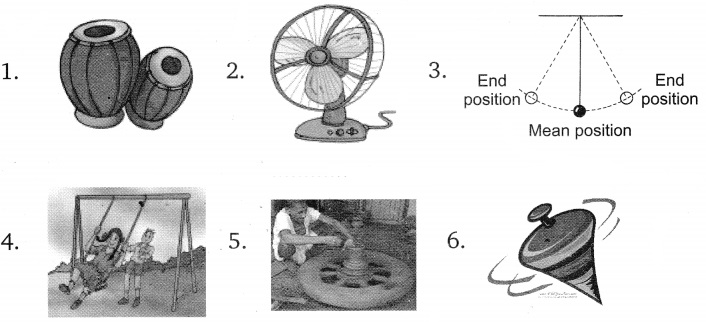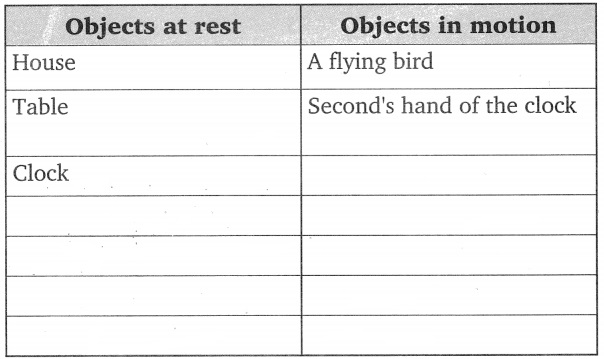Grade 6 Motion and Measurement of Distances Worksheets

A. Answer the following questions in short:

1. Give two examples each of modes of transport used on land, water and air.
2. Why can a pace or a foots tep not be used as a standard unit of length?
3. Arrange the following lengths in their increasing magnitude:
1 metre, 1 centimetre, 1 kilometre, 1 millimetre
4. The height of a person is 1.65 m. Express it into cm and mm.
5. The distance between Radha’s home and her school is 3250 m. Express this’ distance into km.
6. While measuring the length of a knitting needle, the reading of the scale at one end is 3.0 cm and at the other end is 33.1 cm. What is the length of the needle?
7. Write the similarities and differences between the motion of a bicycle and a ceiling fan that has been switched on.
8. Why could you not use an elastic measuring tape to measure distance? What would be some of the problems you would meet in telling someone about a distance you measured with an elastic tape?
9. Give two examples of periodic motion.
10. What do you mean by the SI units? Give examples of some units.
11. Name two common devices used for measuring length.
12. Why do we need to know about the distance?
13. When is a body said to be at rest?

B. Fill in the Blanks:

1. One metre is ………………………. cm.
2. Five kilometre is …………………. m.
3. Motion of a child on a swing is ………………………. .
4. Motion of the needle of a sewing machine is ……………. .
5. Motion of wheel of a bicycle is ……………… .

C. Tick (✓) the Correct Option:

1. A kite flying in the sky has motion of the type :
(a) linear
(b) random
(c) curvilinear
(d) periodic

2. Which of the following shows no change in direction of the moving body?
(a) Curvilinear motion
(b) Oscillatory motion
(c) Rectilinear motion
(d) Revolution

3. The S.I. Unit of length is:
(a) millimetre
(b) metre
(c) kilometre
(d) none of these

4. Which of the following was not used by ancient person for the measurement of length?
(a) Foot
(b) Metre scale
(c) Cubit
(d) Handspan

5. The motion of a pendulum is:
(a) periodic
(b) circular
(c) curvilinear
(d) rotational

D. State True or False:

1. Centimetre is the S.I. unit of length. ………………..
2. The blades of a flying helicopter have different kinds of motion. ………………….
3. A ceiling fan as a whole is at rest when it is on. ………………
4. The motion of the needle of a sewing machine is oscillatory. ……………….
5. Circular motion is also called revolution. ……………..

E. Match the following:

 ‘A’ ‘B’ 1. A ball thrown upward at an angle is the example of a. kilometre 2. Cloth is measured in b. rectilinear motion 3. The motion of potter’s wheel is the example of c. curvilinear motion 4. The distance from Delhi to Mumbai is measured in d. metre 5. A car moving on a straight line is the example of e. rotatory motion

F. Observe the following diagrams and write the type of motion of these objects exhibit while in action or being played.G. Measure the height of your classmate using handspan and then by using a metre scale. For this, ask your classmate to stand with his back against a wall. Make a mark on the wall exactly above his head. Now, measure the distance from the floor to this mark on the wall with your handspan and then with a metre scale. Let all other students measure this length in a similar way. Record all observations in Table given below:

Measurement of height:H. Think of some objects you have seen recently. List them in Table given below:

Objects in rest and motion: